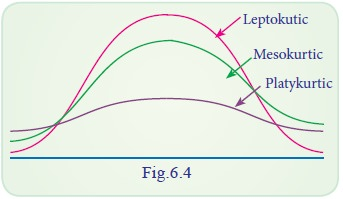Home | | Statistics 11th std | Skewness and Kurtosis

# Skewness and Kurtosis

There are two other comparable characteristics called skewness and kurtosis that help us to understand a distribution.

SKEWNESS AND KURTOSIS

There are two other comparable characteristics called skewness and kurtosis that help us to understand a distribution.

## Skewness

Skewness means ‘lack of symmetry’. We study skewness to have an idea about the shape of the curve drawn from the given data. When the data set is not a symmetrical distribution, it is called a skewed distribution and such a distribution could either be positively skewed or negatively skewed.The concept of skewness will be clear from the following three diagrams showing a symmetrical distribution, a positively skewed distribution and negatively skewed distribution.

We can see the symmetricity from the following diagram.

### (a) Symmetrical Distribution

It is clear from the diagram below that in a symmetrical distribution the values of mean, median and mode coincide. The spread of the frequencies is the same on both sides of the centre point of the curve.### (b) Positively Skewed Distribution

In the positively skewed distribution the value of the mean is maximum and that of mode is least – the median lies in between the two. In the positively skewed distribution the frequencies are spread out over a greater range of values on the high-value end of the curve (the right-hand side) than they are on the low value end. For a positively skewed distribution, Mean>Median> Mode### (c) Negatively skewed distribution

In a negatively skewed distribution the value of mode is maximum and that of mean least-the median lies in between the two. In the negatively skewed distribution the position is reversed, i.e., the excess tail is on the left-hand side.It should be noted that in moderately symmetrical distribution the interval between the mean and the median is approximately one-third of the interval between the mean and the mode. It is this relationship which provides a means of measuring the degree of skewness.

### d. Some important Measures of Skewness

(i) Karl-Person coefficient of skewness

(ii) Bowley’s coefficient of skewness

(iii) Coefficient of skewness based on moments

### (i) Karl-Person coefficient of skewness

According to  Karl-Person the absolute measure of skewness = mean – Mode.

Karl-Pearson coefficient of skewness = Mean – Mode / S.D

### Example 6.16

From the known data, mean = 7.35, mode=8 and Variance = 1.69 then find the Karl-Pearson coefficient of skewness.

### Solution:### (ii) Bowley’s coefficient of skewness

In Karl Pearson method of measuring skewness the whole of the series is needed. Prof. Bowley has suggested a formula based on position of quartiles. In symmetric distribution quartiles will be equidistance from the median. Q2Q1 = Q3Q2 , but in skewed distributions it may not happen. Hence

Bowley’s coefficient of skewness (SK)  =### Example 6.17

If Q1 = 40, Q2=50, Q3=60, Bowley’s coefficient of skewness

### Solution:### (iii) Measure of skewness based on Moments

The Measure of skewness based on moments is denoted by β1 and is given by### Example 6.18

Find β1 for the following data μ1=0, μ2=8.76, μ3=-2.91

### Solution:## Kurtosis

Kurtosis in Greek means ‘bulginess’. In statistics kurtosis refers to the degree of flatness or peakedness in the region about the mode of a frequency curve. The degree of kurtosis of distribution is measured relative to the peakedness of normal curve. In other words, measures of kurtosis tell us the extent of which a distribution is more peaked or flat-topped than the normal curve.

The following diagram illustrates the shape of three different curves mentioned below:

If a curve is more peaked than the  normal curve, it is called ‘leptokurtic’. In such a case items are more closely bunched around the mode. On the other hand if a curve is more flat-topped than the normal curve, it is called ‘platykurtic’. The bell shaped normal curve itself is know as ‘mesokurtic’. We can find how much the frequency curve is flatter than the normal curve using measure of kurtosis.### Measures of Kurtosis

The most important measure of kurtosis is the value of the coefficient. It is defined as: coefficient of kurtosis β24 / μ22### Example 6.19

Find the value of β2 for the following data μ1 = 0, μ2 = 4, μ3 = 0, μ4 = 37.6.

### Solution:Tags : Statistics , 11th Statistics : Chapter 6 : Measures of Dispersion
Study Material, Lecturing Notes, Assignment, Reference, Wiki description explanation, brief detail
11th Statistics : Chapter 6 : Measures of Dispersion : Skewness and Kurtosis | Statistics